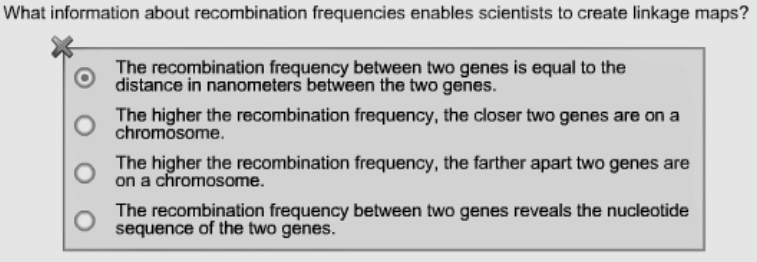# Problem: What information about recombination frequencies enables scientists to create linkage maps?A. The recombination frequency between two genes is equal to the distance in nanometers between the two genes.B. The higher the recombination frequency, the closer two genes are on a chromosome.C. The higher the recombination frequency, the farther apart two genes are on a chromosome.D. The recombination frequency between two genes reveals the nucleotide sequence of the two genes.

###### FREE Expert Solution

A genetic map based on recombination frequencies is known as the linkage map. This is called such because it tries to describe whether a set of genes within the same chromosome are linked or not. If genes are not linked, since there are two sets of chromosomes that serve as the source of the alleles for expression, the chances that one of them would be expressed is 50%. That is the maximum value of probability that both traits are expressed.###### Problem Details

A. The recombination frequency between two genes is equal to the distance in nanometers between the two genes.

B. The higher the recombination frequency, the closer two genes are on a chromosome.

C. The higher the recombination frequency, the farther apart two genes are on a chromosome.

D. The recombination frequency between two genes reveals the nucleotide sequence of the two genes.